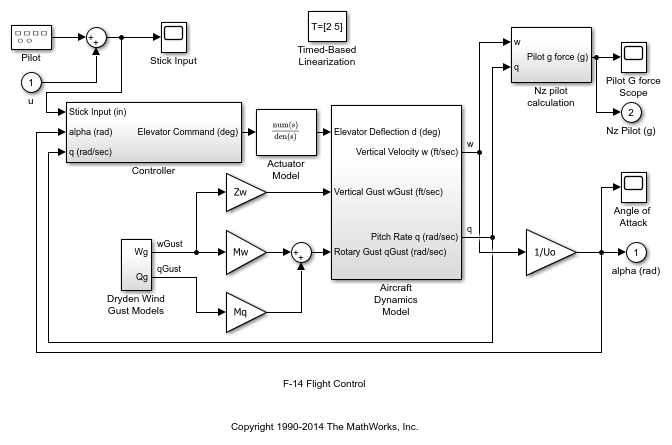# Generate Linear Models at Predetermined Times

This example shows how to use the Timed-Based Linearization block to generate linear models at predetermined times.

In this model, the Linearization time of the Timed-Based Linearization block is set to [2 5]. On the Data Import/Export pane of the Model Configuration Parameters dialog box, the `States` and `Time` check boxes are selected. These settings enable you to get the states of the model at the simulation times of 2 and 5 seconds.After simulating the model, the following variables appear in the MATLAB workspace:

• ex_f14_linearization_Timed_Based_Linearization

• tout

• xout

To get the indices to the operating point times, execute the following command:

```ind1 = find(ex_f14_linearization_Timed_Based_Linearization(1).OperPoint.t==tout); ```

To get the state vectors at the operating points, execute the following command:

```ind2 = find(ex_f14_linearization_Timed_Based_Linearization(1).OperPoint.t==tout); ```